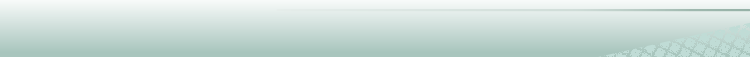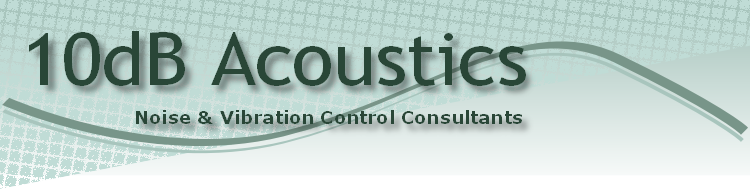﻿ What is a decibelWhat is a decibel (dB)?

The decibel is a logarithmic unit which is used in a number of scientific disciplines. In all cases it is used to compare some quantity with some reference value. Usually the reference value is the smallest likely value of the quantity. Sometimes it can be an approximate average value. In acoustics the decibel is most often used to compare sound pressure, in air, with a reference pressure. References for sound intensity, sound power and sound pressure in water are amongst others which are also commonly in use.

Reference sound pressure (in air) = 0.00002 = 2x10-5 Pa (rms)

Reference sound intensity = 0.000000000001 = 10-12 W/m^2

Reference sound power = 0.000000000001 = 10-12 W

Reference sound pressure (water) = 0.000001 = 10-6 Pa

Acousticians use the dB scale for the following reasons:

• Quantities of interest often exhibit such huge ranges of variation that a dB scale is more convenient than a linear scale. For example, sound pressure radiated by a submarine may vary by eight orders of magnitude depending on direction.
• The human ear interprets loudness on a scale much closer to a logarithmic scale than a linear scale.

How is sound measured?

A sound level meter is the principal instrument for general noise measurement. The indication on a sound level meter (aside from weighting considerations) indicates the sound pressure, p, as a level referenced to 0.00002 Pa. Sound Pressure Level = 20 x log (p/0.00002) dB.

Peak levels are occasionally quoted. During any given time interval peak levels will be numerically greater, and often much greater than the (rms) sound pressure level.